2019-03-01 20:59:51 m0_37180911 阅读数 116
• ###### 第三章：深度学习训练与优化

本章介绍深度学习模型的训练和优化

1011 人正在学习 去看看 CSDN就业班

# 优化算法

## 最小批量梯度下降

learning rate选择太小收敛速度慢，如果太大，loss function就会在极小值处不停地震荡甚至偏离。在鞍点，BGD会停止不动，如果是MBGD或SGD，会发生震荡。如果是mini-batch或者SGD，每次找到的梯度都是不同的，就会发生震荡，来回跳动。

0.9

2

## RMSProp

Root Mean Square Prop

Momentum+RMSProp的微调版本

# 批量归一化

BN就是把输入值尽可能地归一化在激活函数这一狭窄区域。：减低网络地性能。

2018-04-15 19:53:25 shwan_ma 阅读数 715
• ###### 第三章：深度学习训练与优化

本章介绍深度学习模型的训练和优化

1011 人正在学习 去看看 CSDN就业班

SGD算法是一种非常经典的优化算法，其衍生于批梯度下降（BGD）算法。由于 BGD算法需要对整个训练集进行计算梯度，然后再进行更新梯度，其带来的开销是非常大的。因此 用一个mini-batch去更新梯度可以有效减少计算开销，加速收敛，还不容易陷入鞍点。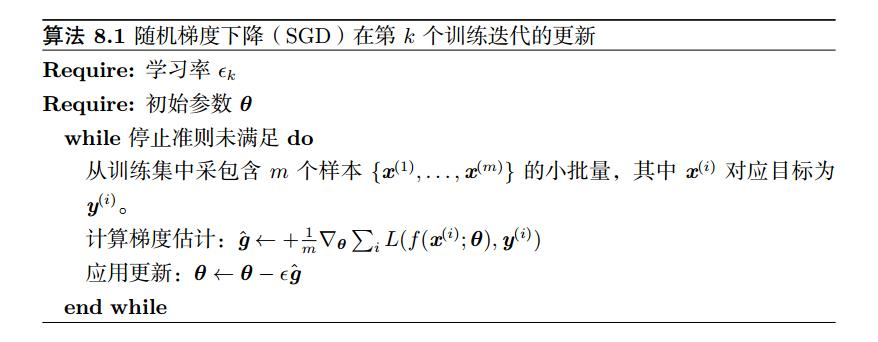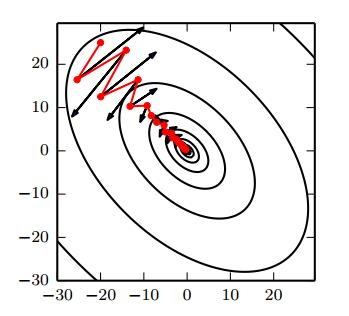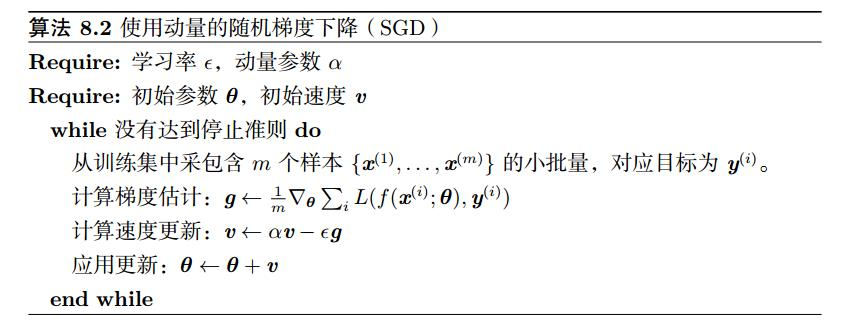Nesterov动量 认为，竟然梯度方向是当年mini-batch样本更新的方向说了不算，那为什么不按照原来动量的方向去更新看看，然后在计算更新后的梯度，从而进行校正呢？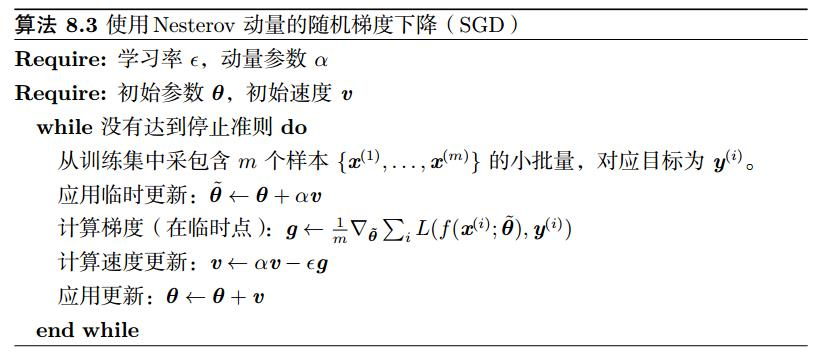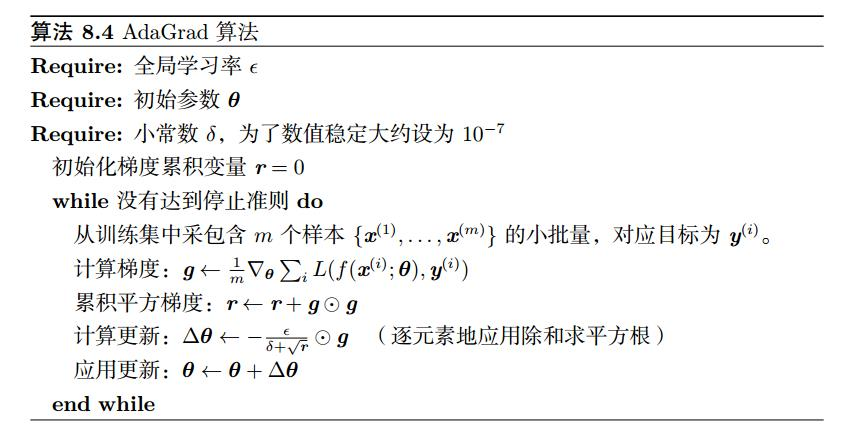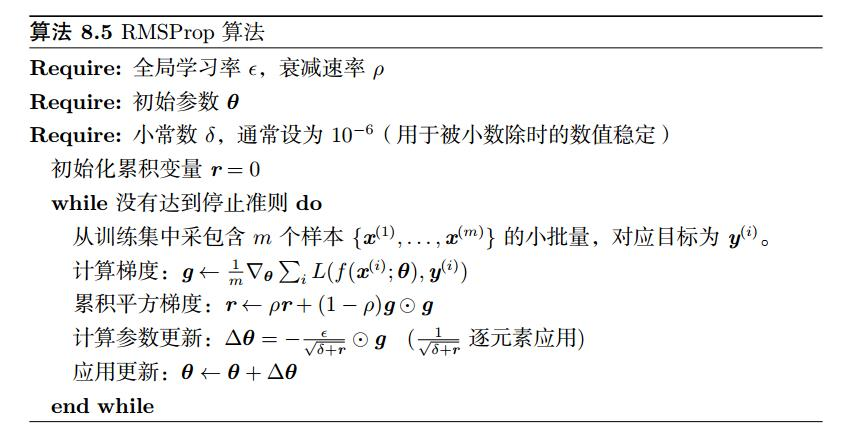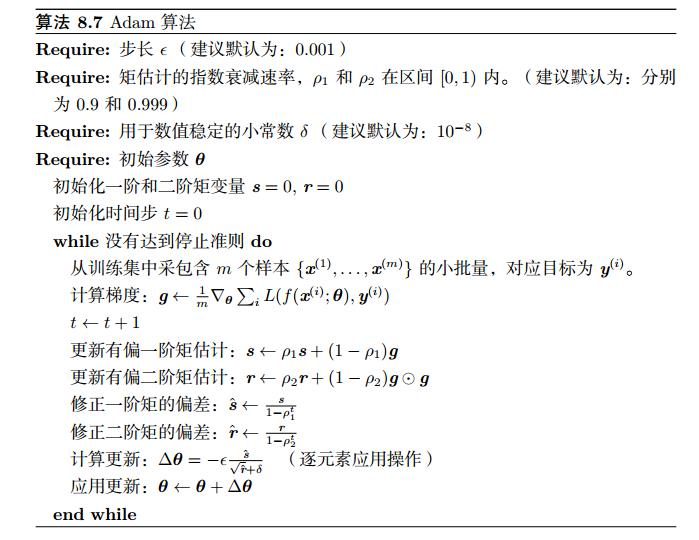================================================================================

V <= beta * V + (1-beta) * theta
V可以近似看作为 1/(1-beta) 个数据的平均值， 如beta=0.1，则近似为10个数据的平均值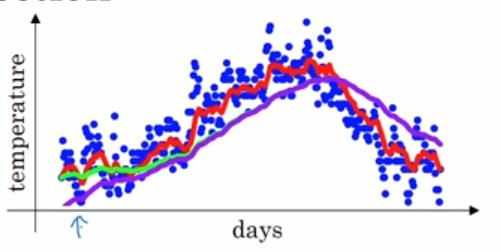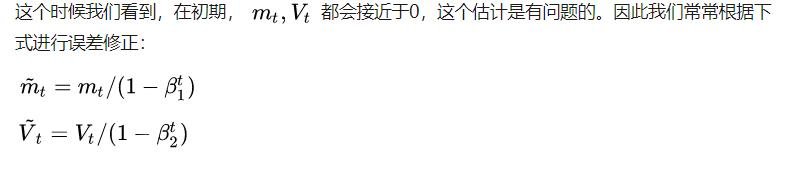2018-03-15 17:24:29 u013498583 阅读数 286
• ###### 第三章：深度学习训练与优化

本章介绍深度学习模型的训练和优化

1011 人正在学习 去看看 CSDN就业班

SGD

SGD就是每一次迭代计算mini-batch的梯度，然后对参数进行更新，是最常见的优化方法了。即：

${g}_{t}={\mathrm{\nabla }}_{{\theta }_{t-1}}f\left({\theta }_{t-1}\right)$

$\mathrm{\Delta }{\theta }_{t}=-\eta \ast {g}_{t}$

SGD完全依赖于当前batch的梯度，所以\eta可理解为允许当前batch的梯度多大程度影响参数更新

- 对所有的参数更新使用同样的learning rate。对于稀疏数据或者特征，有时我们可能想更新快一些对于不经常出现的特征，对于常出现的特征更新慢一些，这时候SGD就不太能满足要求了

SGD容易收敛到局部最优，并且在某些情况下可能被困在鞍点【原来写的是“容易困于鞍点”，经查阅论文发现，其实在合适的初始化和step size的情况下，鞍点的影响并没这么大。感谢@冰橙的指正】

Momentum
momentum是模拟物理里动量的概念，积累之前的动量来替代真正的梯度。公式如下：

${m}_{t}=\mu \ast {m}_{t-1}+{g}_{t}$

$\mathrm{\Delta }{\theta }_{t}=-\eta \ast {m}_{t}$

Nesterov
nesterov项在梯度更新时做一个校正，避免前进太快，同时提高灵敏度。

$\mathrm{\Delta }{\theta }_{t}=-\eta \ast \mu \ast {m}_{t-1}-\eta \ast {g}_{t}$

${g}_{t}={\mathrm{\nabla }}_{{\theta }_{t-1}}f\left({\theta }_{t-1}-\eta \ast \mu \ast {m}_{t-1}\right)$

${m}_{t}=\mu \ast {m}_{t-1}+{g}_{t}$

$\mathrm{\Delta }{\theta }_{t}=-\eta \ast {m}_{t}$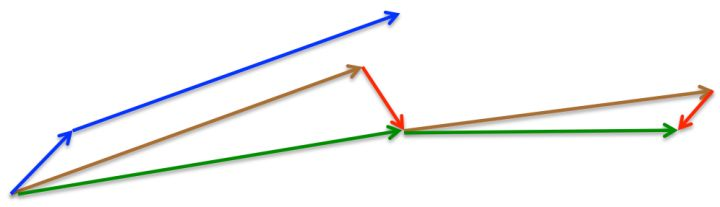momentum首先计算一个梯度(短的蓝色向量)，然后在加速更新梯度的方向进行一个大的跳跃(长的蓝色向量)，nesterov项首先在之前加速的梯度方向进行一个大的跳跃(棕色向量)，计算梯度然后进行校正(绿色梯向量)

${n}_{t}={n}_{t-1}+{g}_{t}^{2}$

$\mathrm{\Delta }{\theta }_{t}=-\frac{\eta }{\sqrt{{n}_{t}+ϵ}}\ast {g}_{t}$

$\eta$$\eta$设置过大的话，会使$regularizer$$regularizer$过于敏感，对梯度的调节太大

${n}_{t}=\nu \ast {n}_{t-1}+\left(1-\nu \right)\ast {g}_{t}^{2}$

$\mathrm{\Delta }{\theta }_{t}=-\frac{\eta }{\sqrt{{n}_{t}+ϵ}}\ast {g}_{t}$

$E|{g}^{2}{|}_{t}=\rho \ast E|{g}^{2}{|}_{t-1}+\left(1-\rho \right)\ast {g}_{t}^{2}$

$\mathrm{\Delta }{x}_{t}=-\frac{\sqrt{\sum _{r=1}^{t-1}\mathrm{\Delta }{x}_{r}}}{\sqrt{E|{g}^{2}{|}_{t}+ϵ}}$

RMSprop

$\rho =0.5$$\rho=0.5$时，$E|{g}^{2}{|}_{t}=\rho \ast E|{g}^{2}{|}_{t-1}+\left(1-\rho \right)\ast {g}_{t}^{2}$$E|g^2|_t=\rho*E|g^2|_{t-1}+(1-\rho)*g_t^2$就变为了求梯度平方和的平均数。

$RMS|g{|}_{t}=\sqrt{E|{g}^{2}{|}_{t}+ϵ}$$RMS|g|_t=\sqrt{E|g^2|_t+\epsilon}$

$\mathrm{\Delta }{x}_{t}=-\frac{\eta }{RMS|g{|}_{t}}\ast {g}_{t}$$\Delta{x_t}=-\frac{\eta}{RMS|g|_t}*g_t$

- 对于RNN效果很好

${m}_{t}=\mu \ast {m}_{t-1}+\left(1-\mu \right)\ast {g}_{t}$

${n}_{t}=\nu \ast {n}_{t-1}+\left(1-\nu \right)\ast {g}_{t}^{2}$

$\stackrel{^}{{m}_{t}}=\frac{{m}_{t}}{1-{\mu }^{t}}$

$\stackrel{^}{{n}_{t}}=\frac{{n}_{t}}{1-{\nu }^{t}}$

$\mathrm{\Delta }{\theta }_{t}=-\frac{\stackrel{^}{{m}_{t}}}{\sqrt{\stackrel{^}{{n}_{t}}}+ϵ}\ast \eta$

- 适用于大数据集和高维空间

${n}_{t}=max\left(\nu \ast {n}_{t-1},|{g}_{t}|\right)$

$\mathrm{\Delta }x=-\frac{\stackrel{^}{{m}_{t}}}{{n}_{t}+ϵ}\ast \eta$

$\stackrel{^}{{g}_{t}}=\frac{{g}_{t}}{1-{\mathrm{\Pi }}_{i=1}^{t}{\mu }_{i}}$

${m}_{t}={\mu }_{t}\ast {m}_{t-1}+\left(1-{\mu }_{t}\right)\ast {g}_{t}$

$\stackrel{^}{{m}_{t}}=\frac{{m}_{t}}{1-{\mathrm{\Pi }}_{i=1}^{t+1}{\mu }_{i}}$

${n}_{t}=\nu \ast {n}_{t-1}+\left(1-\nu \right)\ast {g}_{t}^{2}$

$\stackrel{^}{{n}_{t}}=\frac{{n}_{t}}{1-{\nu }^{t}}\overline{{m}_{t}}=\left(1-{\mu }_{t}\right)\ast \stackrel{^}{{g}_{t}}+{\mu }_{t+1}\ast \stackrel{^}{{m}_{t}}$

$\mathrm{\Delta }{\theta }_{t}=-\eta \ast \frac{\overline{{m}_{t}}}{\sqrt{\stackrel{^}{{n}_{t}}}+ϵ}$

SGD通常训练时间更长，但是在好的初始化和学习率调度方案的情况下，结果更可靠

2017-10-27 00:03:02 xiaocui235 阅读数 1817
• ###### 第三章：深度学习训练与优化

本章介绍深度学习模型的训练和优化

1011 人正在学习 去看看 CSDN就业班

2.1 平均加权（Exponentially Weight Average）
2.2 动量（Momentum）梯度下降法
2.3 RMSprop算法
2.5 学习率衰减

## 一、Mini-batch梯度下降batch梯度下降时，J成本函数随迭代次数下降，而mini-batch的成本函数整体趋势是下降的，但是有噪声产生。## 二、优化算法

### 2.1 平均加权（Exponentially Weight Average）

S_t=

{Y1,βYt+(1β)St1,t=1t>1β$\beta$=0.9时，相当于考虑了10天的温度。如图中红线所示。
β$\beta$=0.98时，相当于考虑了50天的温度。如图中绿线所示，比红线更平滑，但是会有延迟。
β$\beta$ =0.95时，相当于考虑了2天的温度。数据较少，波动较大，会有异常值。但是这个曲线能更快适应温度变化。### 2.2 动量（Momentum）梯度下降法On iteration t:
Compute dW,db on the current mini-batch

VdW=βVdW+(1β)dW

Vdb=βVdb+(1β)db

W=WαVdW,b=bαVdb

### 2.3 RMSprop算法

RMSprop全称是root mean square prop算法，是另一种加速梯度下降的方法。为了便于理解，我们假设纵轴代表参数b，横轴为W。与Momentum类似，我们想减慢纵轴的变化，同时加快横轴的学习，至少不是减缓横轴方向的学习。
On iteration t:
Compute dW,db on the current mini-batch

SdW=βSdW+(1β)dW2

Sdb=βSdb+(1β)db2

W=WαdWSdW,b=bαdbSdb

W=WαdWSdW+ϵ,b=bαdbSdb+ϵRMSprop和Adam优化算法是少有的经受的考验的两种算法。已被证明适用于不同的深度学习结构，并且能够很好地解决许多问题。Adam算法基本是Momentum和RMSprop的结合，相当于Momentum更新了β1$\beta_1$ ，RMSprop更新了β2$\beta_2$。首先初始化:

VdW=0,Vdb=0,SdW=0,Sdb=0

On iteration t:
Compute dW,db on the current mini-batch
VdW=β1VdW+(1β1)dW

Vdb=β1Vdb+(1β1)db

SdW=β2SdW+(1β2)dW2

Sdb=β2Sdb+(1β2)db2

W=WαdWSdW,b=bαdbSdb

VcorrecteddW=VdW1βt1,Vcorrecteddb=Vdb1βt1

ScorrecteddW=SdW1βt2,Scorrecteddb=Sdb1βt2

W=WαVcorrecteddWSdW+ϵ,b=bαVcorrecteddbSdb+ϵ

### 2.5 学习率衰减α=11+decayrateepochnumα0

decay_rate是衰减率，epoch_num是代数， α0$\alpha_0$是初始学习率。其中，在实际应用中，我们可以多尝试不同的衰减率和初始学习率，调整到合适的值。此外还有其它的学习率衰减公式：
α=0.95epochnumα0

α=kepochnumα0k

α=ktα0tminibatcht## 三、局部最优## 参考资料

2018-03-13 09:23:47 qq_28031525 阅读数 3034
• ###### 第三章：深度学习训练与优化

本章介绍深度学习模型的训练和优化

1011 人正在学习 去看看 CSDN就业班

本文基于目前深度学习中使用较多的优化学习算法进行总结。

#### 1 深度学习中的优化算法

优化算法之前讨论两个问题：
(1) 局部最小值问题
在深度学习中，最优化问题其实并不容易困在局部最小值点。在高维度空间时（如20000维），局部最小值点需要在所有维度上都取得最小值，概率为${2}^{-20000}$$2^{-20000}$，相反更容易困在鞍点处。
(2) 指数加权(移动)平均（Exponentially Weighted (moving) average）
在数据抖动较大的情况下，利用之前的整体变化趋势预测当前值。例如预测今天的气温，可以对之前一段时间的气温做平滑处理，即指数加权移动平均，一般形式为:

${v}_{t}=\beta {v}_{t-1}+\left(1-\beta \right){\theta }_{t}$

其中数据${v}_{t}$$v_{t}$利用了前$\frac{1}{1-\beta }$$\frac{1}{1-\beta }$天的整体趋势平均。为什么是前$\frac{1}{1-\beta }$$\frac{1}{1-\beta }$时刻的的指数平均可以从上图看出。当我们迭代带入数值计算时，一般认为当$\left(t-n\right)$$(t-n)$时刻的权重小于$t$$t$时刻的$e$$e$分之一（$\left(1-\epsilon {\right)}^{\frac{1}{\epsilon }}\approx \frac{1}{e}$$(1-\varepsilon )^{\frac{1}{\varepsilon }}\approx \frac{1}{e}$，取$\beta =\left(1-\epsilon \right)$$\beta=(1-\varepsilon )$，参数变换即${\beta }^{\frac{1}{1-\beta }}\approx \frac{1}{e}$$\beta ^{\frac{1}{1-\beta }}\approx \frac{1}{e}$）时，就不再认为$\left(t-n\right)$$(t-n)$时刻前的数据参与平均。那么可以看到当$\beta$$\beta$为0.9的时候，指数系数为10，也即${\beta }^{\frac{1}{1-\beta }}={0.9}^{10}$$\beta ^{\frac{1}{1-\beta }}=0.9^{10}$，共10个时刻的数据参与平均，当$\beta$$\beta$为0.98时，指数系数为50，也即${\beta }^{\frac{1}{1-\beta }}={0.98}^{50}$$\beta ^{\frac{1}{1-\beta }}=0.98^{50}$，认为共50个时刻的数据参与平均。

##### 1.1 随机梯度下降（SGD）其中，$\eta$$\eta$是学习率，${g}_{t}$$g_{t}$是梯度。SGD完全依赖于当前batch的梯度，所以$\eta$$\eta$可理解为允许当前batch的梯度多大程度影响参数更新。
通常来说，就总训练时间和最终代价而言，最优初始化学习率的效果会好于大约迭代100次后的效果。
缺点：
(1) 选择合适的learning rate比较困难，且对所有的参数更新使用同样的learning rate
(2) SGD容易收敛到局部最优，并且在某些情况下可能被困在鞍点（其实在合适的初始化和step size的情况下，鞍点的影响并没有很大）

##### 1.2 动量（momentum）

动量方法旨在加速学习，特别是在面对小而连续的梯度但是含有很多噪声的时候。动量模拟了物体运动时的惯性，即在更新的时候在一定程度上会考虑之前更新的方向，同时利用当前batch的梯度微调最终的结果。这样则可以在一定程度上增加稳定性，从而更快的学习。特点：
(1) 下降初期时，使用上一次参数更新，当下降方向一致时能够加速学习
(3) 在梯度改变方向时，能减少更新。总体而言，momentum能够在相关方向上加速学习，抑制振荡，从而加速收敛

##### 1.3 Nesterov动量

Nesterov项在梯度更新时做出校正，避免前进太快，同时提高灵敏度。在动量中，我们将其展开为：可以看到，此前积累的动量${m}_{t-1}$$m_{t-1}$并没有直接改变当前梯度${g}_{t}$$g_{t}$，所以Nesterov的改进就是让之前的动量直接影响当前的动量，即：所以，加上Nesterov项后，梯度在大的跳跃后，进行计算对当前梯度进行校正。
Nesterov动量和标准动量的区别在于梯度的计算上。Nesterov动量的梯度计算是在施加当前速度之后。因此，Nesterov动量可以解释为往标准动量方法中添加了一个校正因子。

##### 1.4 自适应学习率算法

在训练开始的时候，我们远离最终的最优值点，需要使用较大的学习率。经过几轮训练之后，我们需要减小训练学习率。
在采用mini-batch梯度下降时，迭代的过程中会伴随有噪音，虽然cost function会持续下降，但是算法收敛的结果是在最小值附近处摆动，而减小学习率，则会使得最后的值在最小值附近，更加接近收敛点。Divide the learning rate of each parameter by the root mean square of its previous derivatives(将每个参数除以之前所有梯度的均方和)。此处，对${n}_{t}$$n_{t}$从t=1开始进行递推形成一个约束项regularizer，$\epsilon$$\varepsilon$保证分母非0。
特点：
(1) 前期${g}_{t}$$g_{t}$较小的时候，regularizer较大，能够放大梯度
(2) 后期${g}_{t}$$g_{t}$较大的时候，regularizer较小，能够约束梯度
(3) 适合处理稀疏梯度。
缺点：
(1) 需要手动设置一个全局的学习率
(2) $\eta$$\eta$设置过大时，会使regularizer过于敏感，对梯度的调节太大特点：
(1) 训练初中期，加速效果不错
(2) 训练后期，反复在局部最小值附近抖动

##### 1.4.3 RMSProp

RMSProp可以看做为Adadalta的一个特例，也即上述式子中$\rho =0.5$$\rho =0.5$，然后再求根的话，就变成RMS（均方根）：随着${\eta }_{t}$$\eta _{t}$在不同范围内（$\frac{1}{1-v}$$\frac{1}{1-v}$）的平均，对于变化较大的值方向能够抑制变化，较小的值方向加速变化，消除摆动加速收敛RMSProp的特点如下，
优点：
(2) 适用于处理非平稳过程（也即过程依赖于时间，采用指数加权平均时对于非平稳过程处理较好）-对于RNN效果较好
缺点：
(1) 仍然依赖于全局学习率

偏置修正(Bias Correction)的效果：
当初始化${v}_{0}=0$$v_{0}=0$时，${v}_{1}=0.98{v}_{0}+0.02{\theta }_{1}$$v_{1}=0.98v_{0}+0.02\theta _{1}$（其中${\theta }_{1}$$\theta _{1}$为当前时刻的值），可以看到${v}_{1}$$v_{1}$远小于当前的值${\theta }_{1}$$\theta _{1}$，接下来${v}_{2}=0.98{v}_{1}+0.02\theta =0.98\ast 0.02{\theta }_{1}+0.02{\theta }_{2}$$v_{2}=0.98v_{1}+0.02\theta =0.98*0.02\theta _{1}+0.02\theta _{2}$，得到的结果${\theta }_{2}$$\theta _{2}$远小于当前时刻的值${\theta }_{2}$$\theta _{2}$，因此在训练初期时与真实值会有较大误差（也即初期时绿色线和紫色线的差别）。
因此采用${v}_{t}=\frac{{v}_{t}}{1-{\beta }^{t}}$$v_{t}=\frac{v_{t}}{1-\beta ^{t}}$时，当t较小时，${v}_{t}$$v_{t}$能得到修正偏差，也即下图右边所示，而当t逐渐变大时，由于$\beta$$\beta$小于1，则偏置效果减弱。通常，$\mu$$\mu$的值可以设置为0.9，$v$$v$的值可以设置为0.999。其中，${m}_{t}$$m _{t}$,${n}_{t}$$n _{t}$分别是梯度一阶矩估计和二阶矩估计，可以看做对期望$E|{g}_{t}|$$E\left | g_{t} \right |$,$E|{g}_{t}^{2}|$$E\left |g_{t}^{2}\right |$的估计，近似为对期望的无偏估计。
特点：
(2) 对内存需求较小（指数加权平均不需要存储大量的值就能平均）
(3) 为不同的参数计算不同的自适应学习率
(4) 适用于大多非凸优化 - 适用于大数据集和高维空间